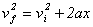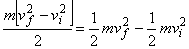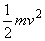# Physics Assignment Help With Work Energy Theorem## Work Energy Theorem

Let us study what physical quantity changes when work is done on a particle. If a constant force F acts through a displacement x, it does work W = Fx = (ma) x on the particle.
Since the acceleration is constant, we can use the equation of kinematicsThus,W =The quantity K =is a scalar and is called the kinetic energy of the particle.

Kinetic energy is the energy that a particle posses by virtue of its motion.
Thus, the equation takes the form
W = Kf - Ki = DK

The work done by a force changes the kinetic energy of the particle. This is called the
Work-Energy Theorem.

### In General

The net work done by the resultant of all the force acting on the particle is equal to the change in kinetic energy of a particle.
Wnet­ = DK

### Important

(i) The kinetic energy of an object is a measure of the amount of work needed to increase its speed from zero to a given value.
(ii) The kinetic energy of a particle is the work it can do on its surroundings in coming to rest.
(iii) Since the velocity and displacement of a particle depend on the frame of reference, the numerical values of the work and the kinetic energy also depend on the frame.

### Assignment Help For Work Energy Theorem

assignmenthelp.net provides best Online Assignment Help service in Physics for all standards. Our Tutor provide their high quality and optimized Tutorial help to fulfill all kind of need of Students.